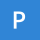Home
IT Knowledge
Inspiration
LanguagesEN

# Create simple animated bar chart in React

3 points
Created by:Paris-Bateman
314

Did you know you don't need much to build an animated bar chart?
In this article, I will try to explain how to get such an effect with React. 📊

Final effect of the post:

The main idea is to create individual bars that are described from 0 to 100%. The bars are arranged sequentially next to each other in a chart, using an example data set
based on the cosine function.

Each change of the dataset describing the chart causes a smooth re-rendering (this happens due to a simple style called `transition`).

The whole effect is achieved with a few lines, most of the effect is actually styles. 🎨

Practical example:

``````// ONLINE-RUNNER:browser;

// import React from 'react';
// import ReactDOM from 'react-dom';

// ---------------------------------

const containerStyle = {
background: '#ffffff',
flex: '1'
};

const spaceStyle = {
background: '#ffffff',
transition: '0.3s'
};

const barStyle = {
background: '#00cc00',
transition: '0.3s'
};

const Bar = ({value}) => {
return (
<div style={containerStyle}>
<div style={{...spaceStyle, height: `\${100 - value}%`}} />
<div style={{...barStyle, height: `\${value}%`}} />
</div>
);
};

const chartStyle = {
width: '400px',
height: '300px',
display: 'flex',
overflow: 'hidden'
};

const Chart = ({data}) => {
return (
<div style={chartStyle}>
{data.map((value, index) => <Bar key={index} value={value} />)}
</div>
);
};

// ---------------------------------

const calculateData = (xOffset) => {
const data = [ ];
for (var x = 0; x < 3.1415; x += 0.1) {
const y = Math.cos(x + xOffset) + 1;
data.push(50 * y);
}
return data;
};

const App = () => {
const [data, setData] = React.useState(() => calculateData(0));
const xOffsets = [0, 0.7853, 1.5707, 2.3559, 3.1415];
return (
<div>
<Chart data={data} />
<br />
<div>
<span>xOffset: </span>
{xOffsets.map(xOffset => {
const handleClick = () => setData(calculateData(xOffset));
return (
<button key={xOffset} onClick={handleClick}>{xOffset}</button>
);
})}
</div>
</div>
);
};

const root = document.querySelector('#root');
ReactDOM.render(<App />, root);``````

What do you think about such a chart? Do you think it would be possible to construct a simple library for different types of charts?

Let me know in the comments! 😊